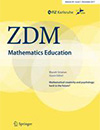# Who’s wrong? Tasks fostering understanding of mathematical relationships in word problems in elementary studentsSavard, A., & Polotskaia, E. (2017). Who’s wrong? Tasks fostering understanding of mathematical relationships in word problems in elementary students. ZDM Mathematics Education, 0(0), 0. https://doi.org/10.1007/s11858-017-0865-5

Abstract: Mathematical relationships are crucial elements to consider for learning mathematics. However, too often students pay more attention to the calculations to be done rather than the reasons for doing them. Relying on the relational paradigm to support elementary school students, we proposed two specially designed tasks to help students recognize and formalize the relationships in additive and multiplicative word problems. These tasks were also designed to have them learn how to represent the relationships using models and to manipulate the mathematical structure of a problem to find the required arithmetic operation. In this paper, we present the task design principles to create mathematically incoherent situation (MIS) tasks and we highlight their implementation with elementary school students. Our findings suggest that students working on MIS tasks really engage in the analysis of the mathematical relationships, which corresponds to the teacher’s intentions in problem-solving activities.